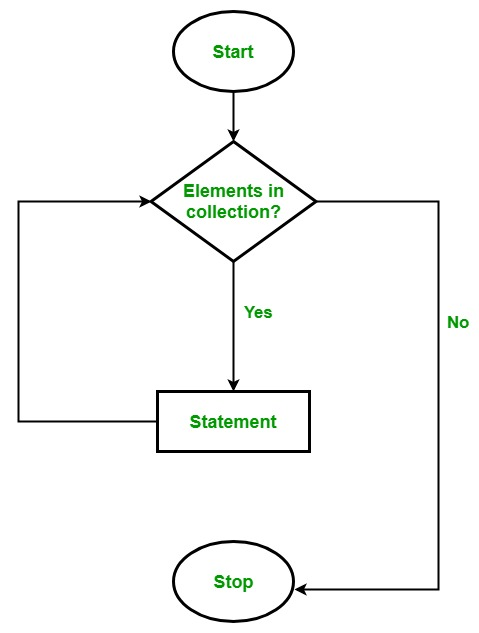# C# | foreach Loop

Prerequisite: Loops in C#

Looping in a programming language is a way to execute a statement or a set of statements multiple numbers of times depending on the result of a condition to be evaluated. The resulting condition should be true to execute statements within loops. The foreach loop is used to iterate over the elements of the collection. The collection may be an array or a list. It executes for each element present in the array.

• It is necessary to enclose the statements of foreach loop in curly braces {}.
• Instead of declaring and initializing a loop counter variable, you declare a variable that is the same type as the base type of the array, followed by a colon, which is then followed by the array name.
• In the loop body, you can use the loop variable you created rather than using an indexed array element.

Syntax:

```foreach(data_type var_name in collection_variable)
{
// statements to be executed
}
```

Flowchart:Example 1:

 `// C# program to illustrate the ` `// use of foreach loop ` `using` `System; ` ` `  `class` `GFG { ` ` `  `    ``// Main Method ` `    ``static` `public` `void` `Main() ` `    ``{ ` ` `  `        ``Console.WriteLine(``"Print array:"``); ` ` `  `        ``// creating an array ` `        ``int``[] a_array = ``new` `int``[] { 1, 2, 3, 4, 5, 6, 7 }; ` ` `  `        ``// foreach loop begin ` `        ``// it will run till the ` `        ``// last element of the array ` `        ``foreach``(``int` `items ``in` `a_array) ` `        ``{ ` `            ``Console.WriteLine(items); ` `        ``} ` `    ``} ` `} `

Output:

```Print array:
1
2
3
4
5
6
7
```

Explanation: foreach loop in above program is equivalent to:

```for(int items = 0; items < a_array.Length; items++)
{
Console.WriteLine(a_array[items]);
}
```

Example 2:

 `// C# program to illustrate  ` `// foreach loop  ` `using` `System; ` ` `  `class` `For_Each      ` `{ ` `     `  `    ``// Main Method ` `    ``public` `static` `void` `Main(String[] arg)  ` `    ``{  ` `        ``{  ` `            ``int``[] marks = { 125, 132, 95, 116, 110 };  ` `             `  `            ``int` `highest_marks = maximum(marks);  ` `             `  `            ``Console.WriteLine(``"The highest score is "` `+ highest_marks);  ` `        ``}  ` `    ``}  ` `     `  `    ``// method to find maximum ` `    ``public` `static` `int` `maximum(``int``[] numbers)  ` `    ``{  ` `        ``int` `maxSoFar = numbers;  ` `         `  `        ``// for each loop  ` `        ``foreach` `(``int` `num ``in` `numbers)  ` `        ``{  ` `            ``if` `(num > maxSoFar)  ` `            ``{  ` `                ``maxSoFar = num;  ` `            ``}  ` `        ``}  ` `    ``return` `maxSoFar;  ` `    ``}  ` `}  `

Output:

```The highest score is 132
```

Limitations of foreach loop:

1. Foreach loops are not appropriate when you want to modify the array:
```foreach(int num in marks)
{
// only changes num not
// the array element
num = num * 2;
}
```
2. Foreach loops do not keep track of index. So we can not obtain array index using ForEach loop
```foreach (int num in numbers)
{
if (num == target)
{
return ???;   // do not know the index of num
}
}
```
3. Foreach only iterates forward over the array in single steps
```// cannot be converted to a foreach loop
for (int i = numbers.Length - 1; i > 0; i--)
{
Console.WriteLine(numbers[i]);
}
```

Difference between for loop and foreach loop:

• for loop executes a statement or a block of statement until the given condition is false. Whereas foreach loop executes a statement or a block of statements for each element present in the array and there is no need to define the minimum or maximum limit.
• In for loop, we iterate the array in both forward and backward directions, e.g from index 0 to 9 and from index 9 to 0. But in the foreach loop, we iterate an array only in the forward direction, not in a backward direction.
• In terms of a variable declaration, foreach loop has five variable declarations whereas for loop only have three variable declarations.
• The foreach loop copies the array and put this copy into the new array for operation. Whereas for loop doesn’t do.

My Personal Notes arrow_drop_upCheck out this Author's contributed articles.

If you like GeeksforGeeks and would like to contribute, you can also write an article using contribute.geeksforgeeks.org or mail your article to contribute@geeksforgeeks.org. See your article appearing on the GeeksforGeeks main page and help other Geeks.

Please Improve this article if you find anything incorrect by clicking on the "Improve Article" button below.

Improved By : GautamNitt

Article Tags :

3

Please write to us at contribute@geeksforgeeks.org to report any issue with the above content.